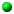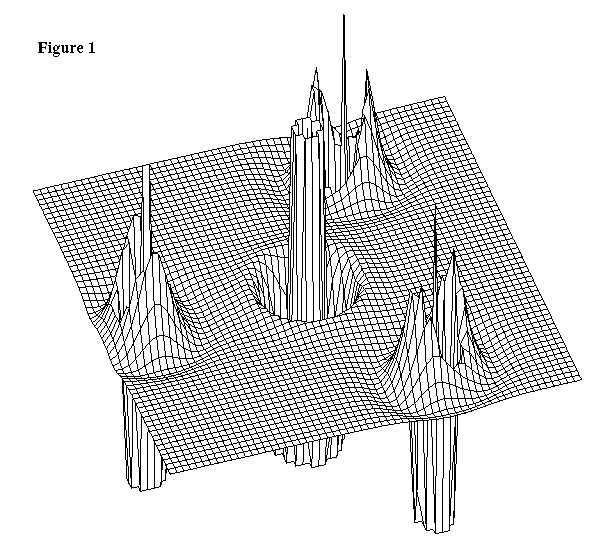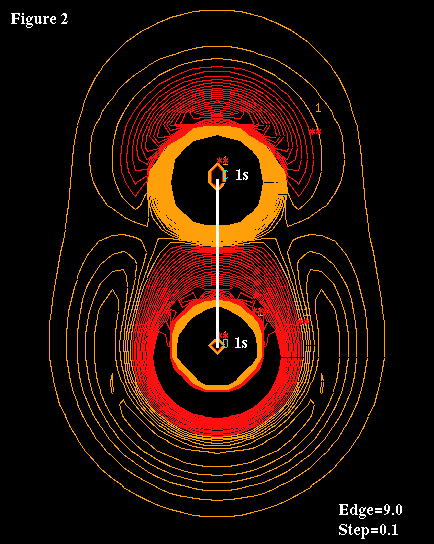The Laplacian of the Electron Density## The Laplacian of the Electron DensityMolden can display the laplacian of the electron density when used with the Ab Initio packages GamessUS/UK and Gaussian. The laplacian of the electron density is the trace of the Hessian matrix. Or in plain(er) language, the sum of the diagonal elements of the second derivative matrix of the density. The laplacian provides interesting information about the chemical bond. This approach has been pioneered by Bader. Have a look at his excellent lecture on The Theory of Atoms in Molecules.
The characteristics of the laplacian can be summerized a follows:In regions where the Laplacian is negative, the potential energy is dominant and the negative charge is concentrated.In regions where the Laplacian is positive, the kinetic energy density dominates and a depletion of negative charge occurs.The Laplacian recovers the shell structure of an atom as a number of alternating shells of charge concentration and charge depletion.A chemical reaction corresponds to the combination of a charge concentration in the valence shell charge concentration of the base with a charge depletion in the valence charge concentration of the acid.

Three commandline flags were introduced to aid the space type of plots of the laplacian.

```     -L   display both neg. and pos. contour in space
plot of the laplacian. Default only the value
specified with "space=value" will be rendered.

-1   use only the lower half of the cubic grid
used for the space type plot

-2   use only the upper half of the cubic grid
used for the space type plot
```

The latter two were introduced to increase the visibility of the inner (enclosed by other surfaces) surfaces.

Figure 1 shows a 3D plot of the Laplacian of ClF3 using a minimal basis set. The keyword Phase was used to show the regions of negative laplacian on top. Notice that Molden automaticaly cuts off high values of the Laplacian for clarity. It was constructed with the following Molden input file:

```    Laplacian 3D plot
file=clf3.out laplacian edge=9.0 phase=-1 postscript 3D
```Figure 2 shows a Euclid plot of the Laplacian of CO using a minimal basis set.Figure 3 shows a VRML plot of the Laplacian of CO using a minimal basis set. It was constructed with -1 molden commandline flag. Clicking on figure 3 will bring up the VRML scene.